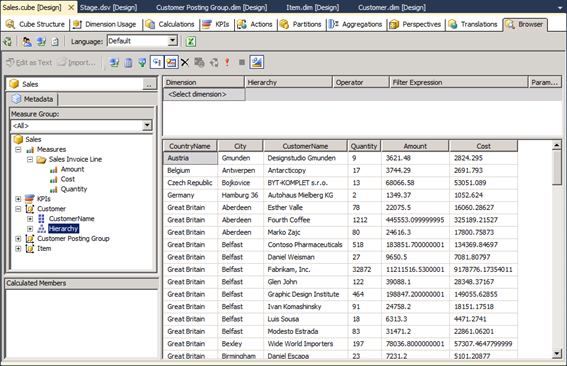# Named Calculations

A named calculation is a SQL expression represented as a calculated column in a table in a Data Source View. This expression appears and behaves as a column in the table. A named calculation lets you extend the relational schema of existing tables or views in a data source view without modifying the tables or views in the underlying data source. For example, a common calculated value derived from the columns of a fact table can be expressed as a single named calculation in the data source view, instead of modifying the underlying database table or creating a view in the underlying database. A named calculation can also be used in place of calculated member in each cube based on the data source view. Named calculations are calculated during processing whereas calculated members are calculated at query time.

### Cost

As we have already seen, the Sales Invoice Line table does contain info about the total Revenue (Amount) per transaction, and we have also applied that as a measure to our Sales cube.

The Sales Invoice Line table does not contain similar info about the total Cost per transaction. But it does contain info about the number of Units Sold (Quantity) and the Cost per Unit (Unit Cost), and based on these two columns we can now derive the desired Cost per transaction:

• Cost = Quantity * Unit Cost

To create this new Named Calculation, we must move to the Data Source View and right-click the header of the Sales Invoice Line table.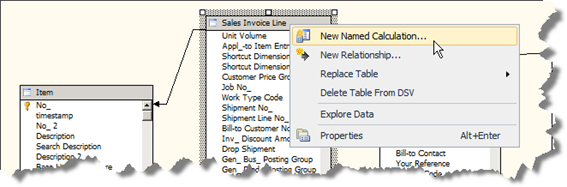The new Named Calculation must contain a name and an expression, and optionally an explanation.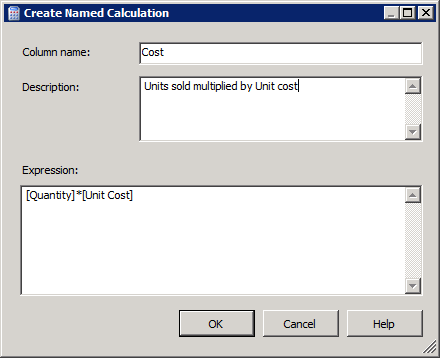The Cost Named Calculation can now be seen at the bottom of the table in the Data Source View:To add this new field as a new measure to our cube, we must first open the cube on the Cube Structure tab and then right-click in the Measures panel to insert a new measure.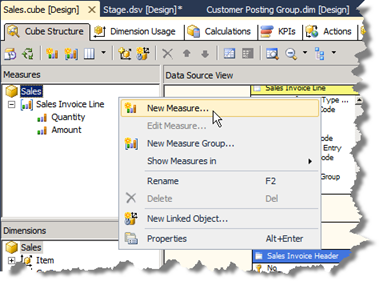The new measure is selected from a list of potential (numerical) columns.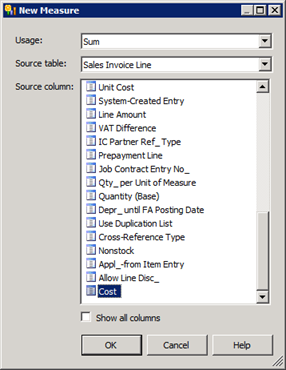After having re-processed and re-connected to the cube, we can see through the cube browser that the Named Calculation is treated like any other measure.